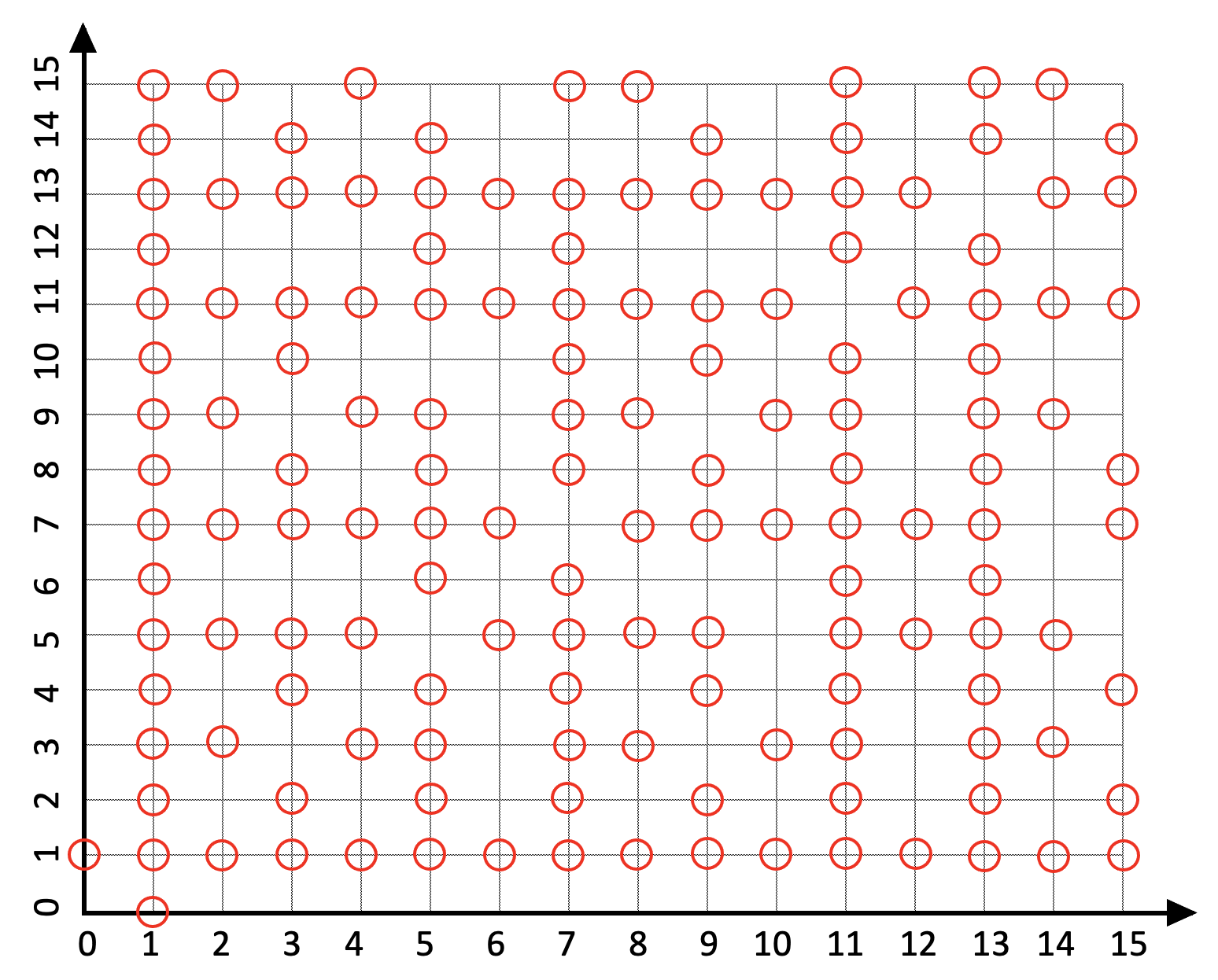# Problem 52764. Easy Sequences 24: Number of Coprime Lattice Points

Given a number 'n', you were tasked to mark and count all coprime lattice points at the first quadrant bounded by the points (0,0), (0,n), (n,n) and (n,0). A coprime lattice point is a point (x,y), where x and y are coprime integers.
For example for n = 15, 145 points would be marked (see figure below).NOTE: One is coprime to all integers including zero. Except for 1, zero is not coprime to any other integer, not even to zero itself.

### Solution Stats

87.5% Correct | 12.5% Incorrect
Last Solution submitted on Jun 17, 2023

### Community Treasure Hunt

Find the treasures in MATLAB Central and discover how the community can help you!

Start Hunting!